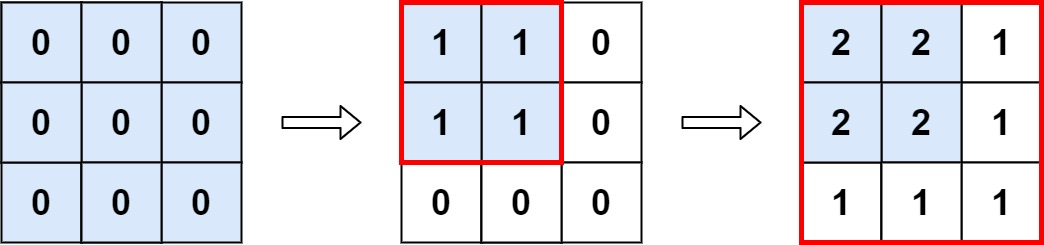| English | 简体中文 |

# 598. Range Addition II

## Description

You are given an m x n matrix M initialized with all 0's and an array of operations ops, where ops[i] = [ai, bi] means M[x][y] should be incremented by one for all 0 <= x < ai and 0 <= y < bi.

Count and return the number of maximum integers in the matrix after performing all the operations.

Example 1:Input: m = 3, n = 3, ops = [[2,2],[3,3]]
Output: 4
Explanation: The maximum integer in M is 2, and there are four of it in M. So return 4.


Example 2:

Input: m = 3, n = 3, ops = [[2,2],[3,3],[3,3],[3,3],[2,2],[3,3],[3,3],[3,3],[2,2],[3,3],[3,3],[3,3]]
Output: 4


Example 3:

Input: m = 3, n = 3, ops = []
Output: 9


Constraints:

• 1 <= m, n <= 4 * 104
• 0 <= ops.length <= 104
• ops[i].length == 2
• 1 <= ai <= m
• 1 <= bi <= n